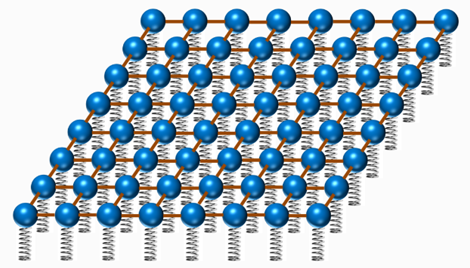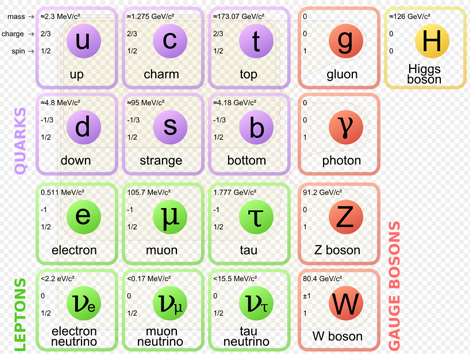# ２２　Quantum Field Theory　#universe #quantum

２２　Quantum Field Theory　#universe #quantum

２２－１　What is Quantum Field?
○Space is quantum fields, particle is the excited quantum field.
※Vacuum (nothing condition) is the quantum fields where the energy is the lowest.
○In quantum field theory, we consider particles（Material fields）as harmonic oscillators and quantize them. Then, as a system of infinite number of harmonic oscillators, the theory is assembled.
※The motion of an object that is connected to a spring and vibrates is called "harmonic oscillation".※Image of Quantum field 

○Quantum field theory combines special relativity theory and quantum mechanics.
・・・The difference between special and general relativity is gravity only. In the world of elementary particles, since the mass is small, the work of gravity is equivalent to almost completely nothing compared to other forces.
○Quantum mechanics is an approximate form of quantum field theory limited to the low energy state.
・Quantum mechanics can not be used for phenomena in which the number of particles changes such as particle generation and extinction.

○Prior to the emergence of string theory, the theory describing the smallest scale was quantum field theory.

２２－２　Field types and particles
１）Gauge field and gauge particle (force mediating particle)
Electromagnetic field ・・・Photon
Weak interaction (causing β decay of neutrons) • • • W boson, Z boson
Strong interaction(Power to combine quarks)・・・Gluon
Gravitational field・・・Graviton

※ Gauge theory(3 + 1 dimension) is the theory underlying the Standard Model.
※As a method to describe gravity, there is a method based on Newton's theory of gravity and general relativity.
※The state with the lowest energy of the string is the gauge particle. ← Superstring Theory

２）Material field and material particles※Under the "quarks and leptons" definition, the elementary and composite particles made of the quarks (in purple) and leptons (in green) would be matter—while the gauge bosons (in red) would not be matter. However, interaction energy inherent to composite particles (for example, gluons involved in neutrons and protons) contribute to the mass of ordinary matter. 

３）Higgs field (scalar field) and Higgs particle・・・Boson →No.２４

２２－３　その他
○Quantum field theory is the fundamental theory of particle physics (describing behavior of elementary particles), nuclear physics, condensed matter physics (describing multi-body theory effects such as critical phenomena and phase transitions).

【References】
1. Brian Skinner”A Children’s Picture-book Introduction to Quantum Field Theory” 20150820
2.Matter - Wikipedia